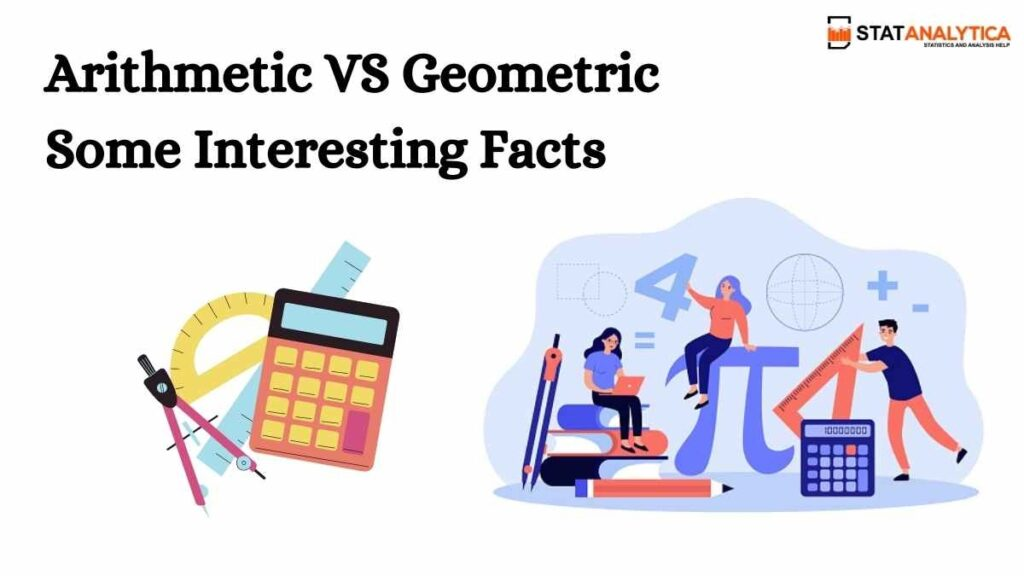# Mathematics## Arithmetic VS Geometric: Some Interesting Facts

For students, mathematics is a very challenging subject, and these concepts “arithmetic vs geometric” also have to deal with infinite numbers. The reason for studying arithmetic or maths is it builds problem-solving skills, and it helps students to think of solutions logically. Arithmetic mainly refers to working with numbers by doing addition, subtraction, multiplication, and …## Best Ever Strategies On How To Be Good At Math by Experts

Many students feel that they are terrible at mathematics and will not improve in maths. They need to know that this is not true. Here is the question that arises is “How to be good at math.” There are lots of people who think that math is tough to understand.  Some people used to search …## List of Top Math Books that are Highly Recommended by Experts

Best math books is the key to success for many students because, before the advancement of the modern age, mathematics had its limits. But, nowadays, it has developed into a vastly diverse topic and they are not up to a limit. The developments in mathematics are still continuous that make great contributions in the technical …## Math Vs Statistics: Top 9 Important Points One Should Know

For students who are confused about which to pursue in Mathematics vs Statistics, this blog will help you. What is the difference between mathematics and statistics? or Math Vs Statistics is very common among students. Several students are not aware of the difference between mathematics and statistics. So, to understand the answer to this question, …## What Are The Top Applications of Calculus in the World

Do you also have this question in your mind? To some extent, it is one of the striking questions that every learner usually faces while learning mathematics. Even the use of mathematics in real life is categorized as an old-age question. Among all the concepts of mathematics, for many of us, calculus is the most …## Geometry vs Trigonometry | What is the Best Between Them

Geometry vs trigonometry, is it important to understand the difference? Yes!! Why?? Because trigonometry is the study of different properties of triangles. On the other hand, geometry helps to study the properties of the geometrical figure. Besides these, there are several differences between geometry and trigonometry that each student must know. This blog will help …## 50+ Mathematics Formula Basic That Students Must Learn In 2021

Do I really need to learn the mathematics formula basic? Isn’t it all about just adding, subtracting, multiplying, and division?  No, it is not!  You need to know the basic math formulas to solve the intermediate and advanced level mathematics problems. Even math is not just restricted to addition and all. It is a lot …## A Powerful Guide on Mathematical Terms for Beginners

Basic mathematical terms and basic mathematical operations go hand in hand. It means both terms and operations are necessary to understand the math problems.  If you do not have any idea regarding the mathematical terms, do not worry, as I have detailed all the necessary terms in this blog. But before that, you all might …## A Definitive Guide on Basic Calculus Formula For The Beginners

Do you know engineering, statistics, physics, economics, and medicine need a basic calculus formula to solve? Moreover, it is the best for creating math and physics models to get the optimal solutions. For instance, calculus basic formulae use in physics concepts like electricity, light, acoustics, motion, astronomy, and more. Apart from this, there are a …## How Statistics Math Problems Look Like & How To Solve Them

Statistics math problems are not only necessary to understand the math question in depth, but it also makes you familiar with real-time things more efficiently. Therefore, it is always important to understand what statistics are and how statistics relate to math.  Don’t have any knowledge of it? No worries!! I will help you know what …